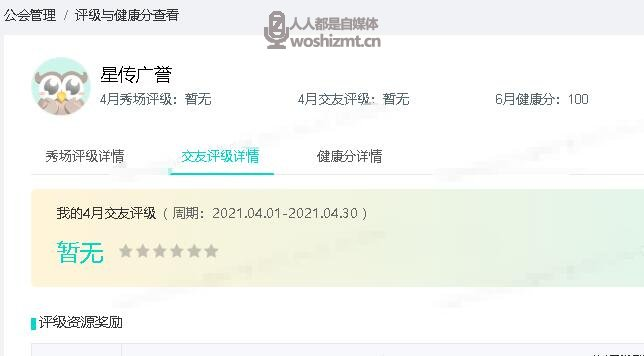# 映客公会评级与健康分规则

8个月前 (12-12) 24次浏览6300050080025072051500350500150540410003003501003603400150250401802150100—1100—-

1. 资源奖励自然月3号，根据公会上月评级自动下发，卡片有效期：本月4号-下月3号

2. 下发资源只能用于推荐秀场直播

6>=100000000>=240>=400>=955>=60000000>=100>=200>=904>=30000000>=80>=100>=853>=10000000>=40>=60>=802>=3000000>=15>=25>=751>=0>=5>=8>=70或

6>=460>=955>=420>=904>=230>=853>=115>=802>=70>=751>=28>=70

1、公会规模取自然月、所有“非交友主播”数据

2、有效主播数：完成18有效天40小时的主播数

3、公会健康分取自然月最后一天分值

4、日均活跃主播数：当月日均单场连续开播20min及以上的主播数（只计算“非交友主播”）

5、如果有两个评级标准时，会分别进行评级，并取其中较高的评级作为最后的评级66080608060805507050705050440504050404032040404030302102020202020151010101010

6>=8000000>=80>=200>=955>=4000000>=40>=150>=904>=2000000>=20>=80>=853>=1000000>=10>=50>=802>=300000>=5>=30>=751>=50000>=1>=10>=75

1、公会规模取自然月数据，只计算所有交友主播的数据

2、公会基准映币流水(元)：等于公会自然月 基准映币总数/10

3、有效厅：当月厅映币收入>=50w映币，且有效直播时长>=180小时；（有效直播时长：单次开播>=20分钟记为一次有效开播）

4、新增有效交友主播：主播首月入驻平台，1）满足有效开播天6天18小时且映币流水>=1000,（有效开播天：当天累计连麦时长>=1小时；有效直播时长：单场直播连麦>=20分钟）或2）映币流水>=100000映票

5、公会健康分取自然月最后一天分值

6、如果有两个评级标准时，会分别进行评级，并取其中较高的评级作为最后的评级